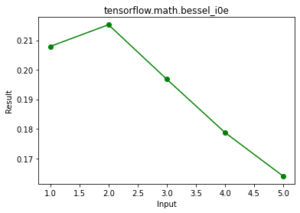# Python – tensorflow.math.bessel_i1e() function

TensorFlow is open-source python library designed by Google to develop Machine Learning models and deep learning  neural networks. bessel_i1e() is function present in tensorflow math module. This function is used to find element wise exponentially scaled first order modified Bessel function.

Syntax: tensorflow.math.bessel_i1e(x, name)

Arguments:

• x: It’s a tensor and the allowed dtypes for this tensor are bfloat16, half, float32, float64.
• name: It’s is an optional argument which is used to give operation name.

Returns: It returns a tensor or sparse tensor depending upon x of same dtype as x.

```bessel_i1e(x) = exp(-abs(x)) bessel_i1(x)

bessel_i1e(x) is faster and numerically stabler than bessel_i1(x).

```

Example 1:

## Python3

 `# importing the library ` `import` `tensorflow as tf ` ` `  `# initializing the input ` `a ``=` `tf.constant([``1``,``2``,``3``,``4``,``5``], dtype ``=` `tf.float64) ` ` `  `# printing the input  ` `print``(``'a: '``,a) ` ` `  `# evaluating the result ` `r ``=` `tf.math.bessel_i1e(a) ` ` `  `# printing the result ` `print``(``"Result: "``,r) `

Output:

```a:  tf.Tensor([1. 2. 3. 4. 5.], shape=(5,), dtype=float64)
Result:  tf.Tensor([0.20791042 0.21526929 0.19682671 0.17875084 0.16397227], shape=(5,), dtype=float64)

```

Example 2: Visualization

## Python3

 `# importing the library ` `import` `tensorflow as tf ` `import` `matplotlib.pyplot as plt  ` ` `  `# initializing the input ` `a ``=` `tf.constant([``1``,``2``,``3``,``4``,``5``], dtype ``=` `tf.float64) ` ` `  `# evaluating the result ` `r ``=` `tf.math.bessel_i1e(a) ` ` `  `#plotting the graph ` `plt.plot(a, r, color ``=` `'green'``, marker ``=` `"o"``)   ` `plt.title(``"tensorflow.math.bessel_i1e"``)   ` `plt.xlabel(``"Input"``)   ` `plt.ylabel(``"Result"``)   ` `plt.show()  `

Output:My Personal Notes arrow_drop_upCheck out this Author's contributed articles.

If you like GeeksforGeeks and would like to contribute, you can also write an article using contribute.geeksforgeeks.org or mail your article to contribute@geeksforgeeks.org. See your article appearing on the GeeksforGeeks main page and help other Geeks.

Please Improve this article if you find anything incorrect by clicking on the "Improve Article" button below.

Article Tags :

Be the First to upvote.

Please write to us at contribute@geeksforgeeks.org to report any issue with the above content.